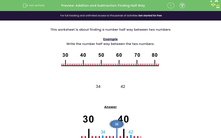# Addition and Subtraction: Finding Half Way

In this worksheet, students find the number half way between two given numbers (from a range between 0 and 100).Key stage:  KS 2

Curriculum topic:   Number: Addition and Subtraction

Curriculum subtopic:   Solve Add/Subtract Problems to 1000

Popular topics:   Subtraction worksheets, Addition worksheets

Difficulty level:#### Worksheet Overview

This worksheet is about finding a number half way between two numbers

Example

Write the number half way between the two numbers.34 42We move in 4 numbers, step by step from 34 and 42 to get to 38.

35, 36, 37, 38, 39, 40, 41

### What is EdPlace?

We're your National Curriculum aligned online education content provider helping each child succeed in English, maths and science from year 1 to GCSE. With an EdPlace account you’ll be able to track and measure progress, helping each child achieve their best. We build confidence and attainment by personalising each child’s learning at a level that suits them.

Get started